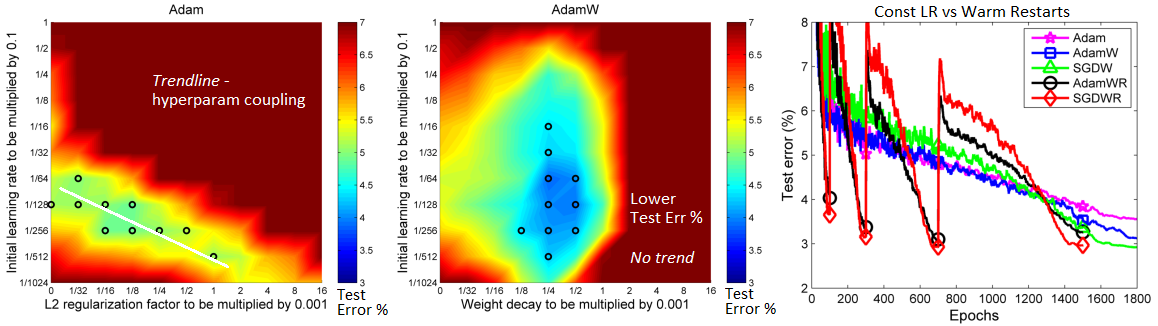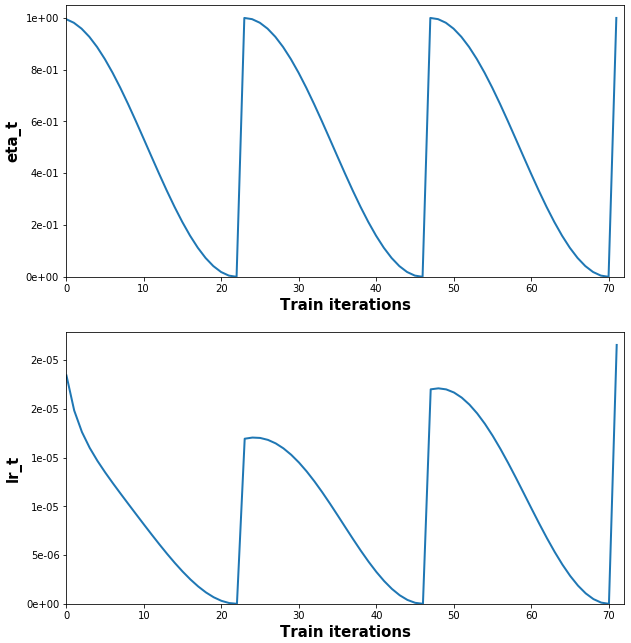Click the “chat” button below for chat support from the developer who created it, or find similar developers for support.OverLordGoldDragon154 Stars20 ForksMIT License50 Commits0 Opened issues#### Description

Keras/TF implementation of AdamW, SGDW, NadamW, Warm Restarts, and Learning Rate multipliers!
?

#### Need anything else?#### Contributors list# 294,747
Python
Keras
Tensorf...
sgd
47 commitsKeras/TF implementation of AdamW, SGDW, NadamW, and Warm Restarts, based on paper Decoupled Weight Decay Regularization - plus Learning Rate Multipliers## Features

• Weight decay fix: decoupling L2 penalty from gradient. Why use?
• Weight decay via L2 penalty yields worse generalization, due to decay not working properly
• Weight decay via L2 penalty leads to a hyperparameter coupling with
`lr`
, complicating search
• Warm restarts (WR): cosine annealing learning rate schedule. Why use?
• Better generalization and faster convergence was shown by authors for various data and model sizes
• LR multipliers: per-layer learning rate multipliers. Why use?
• Pretraining; if adding new layers to pretrained layers, using a global
`lr`
is prone to overfitting

## Installation

`pip install keras-adamw`
or clone repository

## Usage

If using tensorflow.keras imports, set

`import os; os.environ["TF_KERAS"]='1'`
.

### Weight decay

`AdamW(model=model)`

Three methods to set
`weight_decays = {:,}`
:
```# 1. Automatically
Just pass in `model` (`AdamW(model=model)`), and decays will be automatically extracted.
Loss-based penalties (l1, l2, l1_l2) will be zeroed by default, but can be kept via
`zero_penalties=False` (NOT recommended, see Use guidelines).
```
```# 2. Use keras_adamw.utils.py
Dense(.., kernel_regularizer=l2(0)) # set weight decays in layers as usual, but to ZERO
wd_dict = get_weight_decays(model)
# print(wd_dict) to see returned matrix names, note their order
# specify values as (l1, l2) tuples, both for l1_l2 decay
ordered_values = [(0, 1e-3), (1e-4, 2e-4), ..]
weight_decays = fill_dict_in_order(wd_dict, ordered_values)
```
```# 3. Fill manually
model.layers.kernel.name # get name of kernel weight matrix of layer indexed 1
weight_decays.update({'conv1d_0/kernel:0': (1e-4, 0)}) # example
```

### Warm restarts

`AdamW(.., use_cosine_annealing=True, total_iterations=200)`
- refer to Use guidelines below

### LR multipliers

`AdamW(.., lr_multipliers=lr_multipliers)`
- to get,
`{:,}`
:
1. (a) Name every layer to be modified (recommended), e.g.
`Dense(.., name='dense_1')`
- OR
(b) Get every layer name, note which to modify:
`[print(idx,layer.name) for idx,layer in enumerate(model.layers)]`
2. (a)
`lr_multipliers = {'conv1d_0':0.1} # target layer by full name`
- OR
(b)
`lr_multipliers = {'conv1d':0.1}   # target all layers w/ name substring 'conv1d'`

## Example ```python import numpy as np from keras.layers import Input, Dense, LSTM from keras.models import Model from keras.regularizers import l1, l2, l1l2 from kerasadamw import AdamW

ipt = Input(shape=(120, 4)) x = LSTM(60, activation='relu', name='lstm1', kernelregularizer=l1(1e-4), recurrentregularizer=l2(2e-4))(ipt) out = Dense(1, activation='sigmoid', kernelregularizer=l1l2(1e-4, 2e-4))(x) model = Model(ipt, out)

python
lrmultipliers = {'lstm_1': 0.5}

optimizer = AdamW(lr=1e-4, model=model, lrmultipliers=lrmultipliers, usecosineannealing=True, totaliterations=24) model.compile(optimizer, loss='binarycrossentropy')

python
for epoch in range(3):
for iteration in range(24):
x = np.random.rand(10, 120, 4) # dummy data
y = np.random.randint(0, 2, (10, 1)) # dummy labels
loss = model.trainonbatch(x, y)
print("Iter {} loss: {}".format(iteration + 1, "%.3f" % loss))
print("EPOCH {} COMPLETED\n".format(epoch + 1))
```(Full example + plot code, and explanation of

`lr_t`
vs.
`lr`
: example.py)

## Use guidelines

### Weight decay

• Set L2 penalty to ZERO if regularizing a weight via
`weight_decays`
- else the purpose of the 'fix' is largely defeated, and weights will be over-decayed --My recommendation
• `lambda = lambda_norm * sqrt(1/total_iterations)`
--> can be changed; the intent is to scale λ to decouple it from other hyperparams - including (but not limited to), # of epochs & batch size. --Authors (Appendix, pg.1) (A-1)
• `total_iterations_wd`
--> set to normalize over all epochs (or other interval
`!= total_iterations`
) instead of per-WR when using WR; may sometimes yield better results --My note

### Warm restarts

• Done automatically with
`autorestart=True`
, which is the default if
`use_cosine_annealing=True`
; internally sets
`t_cur=0`
after
`total_iterations`
iterations.
• Manually: set
`t_cur = -1`
to restart schedule multiplier (see Example). Can be done at compilation or during training. Non-
`-1`
is also valid, and will start
`eta_t`
at another point on the cosine curve. Details in A-2,3
• `t_cur`
should be set at
`iter == total_iterations - 2`
; explanation here
• Set
`total_iterations`
to the # of expected weight updates for the given restart --Authors (A-1,2)
• `eta_min=0, eta_max=1`
are tunable hyperparameters; e.g., an exponential schedule can be used for
`eta_max`
. If unsure, the defaults were shown to work well in the paper. --Authors
• Save/load optimizer state; WR relies on using the optimizer's update history for effective transitions --Authors (A-2)
```python
# 'total_iterations' general purpose example
def get_total_iterations(restart_idx, num_epochs, iterations_per_epoch):
return num_epochs[restart_idx] * iterations_per_epoch[restart_idx]
get_total_iterations(0, num_epochs=[1,3,5,8], iterations_per_epoch=[240,120,60,30])
```
### Learning rate multipliers
• Best used for pretrained layers - e.g. greedy layer-wise pretraining, or pretraining a feature extractor to a classifier network. Can be a better alternative to freezing layer weights. --My recommendation
• It's often best not to pretrain layers fully (till convergence, or even best obtainable validation score) - as it may inhibit their ability to adapt to newly-added layers. --My recommendation
• The more the layers are pretrained, the lower their fraction of new layers'
`lr`
should be. --My recommendation

## How to cite

Short form:

```@article{OverLordGoldDragon2019keras-adamw,